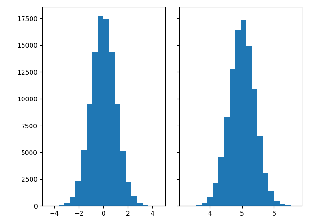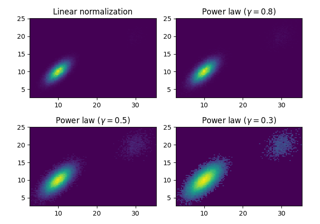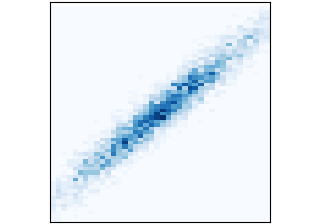# matplotlib.axes.Axes.hist2d#

Axes.hist2d(x, y, bins=10, range=None, density=False, weights=None, cmin=None, cmax=None, *, data=None, **kwargs)[source]#

Make a 2D histogram plot.

Parameters:
x, yarray-like, shape (n, )

Input values

binsNone or int or [int, int] or array-like or [array, array]

The bin specification:

• If int, the number of bins for the two dimensions (nx=ny=bins).

• If `[int, int]`, the number of bins in each dimension (nx, ny = bins).

• If array-like, the bin edges for the two dimensions (x_edges=y_edges=bins).

• If `[array, array]`, the bin edges in each dimension (x_edges, y_edges = bins).

The default value is 10.

rangearray-like shape(2, 2), optional

The leftmost and rightmost edges of the bins along each dimension (if not specified explicitly in the bins parameters): ```[[xmin, xmax], [ymin, ymax]]```. All values outside of this range will be considered outliers and not tallied in the histogram.

densitybool, default: False

Normalize histogram. See the documentation for the density parameter of `hist` for more details.

weightsarray-like, shape (n, ), optional

An array of values w_i weighing each sample (x_i, y_i).

cmin, cmaxfloat, default: None

All bins that has count less than cmin or more than cmax will not be displayed (set to NaN before passing to imshow) and these count values in the return value count histogram will also be set to nan upon return.

Returns:
h2D array

The bi-dimensional histogram of samples x and y. Values in x are histogrammed along the first dimension and values in y are histogrammed along the second dimension.

xedges1D array

The bin edges along the x axis.

yedges1D array

The bin edges along the y axis.

image`QuadMesh`
Other Parameters:
cmapstr or `Colormap`, default: `rcParams["image.cmap"]` (default: `'viridis'`)

The Colormap instance or registered colormap name used to map scalar data to colors.

normstr or `Normalize`, optional

The normalization method used to scale scalar data to the [0, 1] range before mapping to colors using cmap. By default, a linear scaling is used, mapping the lowest value to 0 and the highest to 1.

If given, this can be one of the following:

vmin, vmaxfloat, optional

When using scalar data and no explicit norm, vmin and vmax define the data range that the colormap covers. By default, the colormap covers the complete value range of the supplied data. It is an error to use vmin/vmax when a norm instance is given (but using a `str` norm name together with vmin/vmax is acceptable).

alpha`0 <= scalar <= 1` or `None`, optional

The alpha blending value.

dataindexable object, optional

If given, the following parameters also accept a string `s`, which is interpreted as `data[s]` (unless this raises an exception):

x, y, weights

**kwargs

Additional parameters are passed along to the `pcolormesh` method and `QuadMesh` constructor.

`hist`

1D histogram plotting

`hexbin`

2D histogram with hexagonal bins

Notes

• Currently `hist2d` calculates its own axis limits, and any limits previously set are ignored.

• Rendering the histogram with a logarithmic color scale is accomplished by passing a `colors.LogNorm` instance to the norm keyword argument. Likewise, power-law normalization (similar in effect to gamma correction) can be accomplished with `colors.PowerNorm`.

## Examples using `matplotlib.axes.Axes.hist2d`#Histograms

HistogramsExploring normalizations

Exploring normalizationshist2d(x, y)

hist2d(x, y)